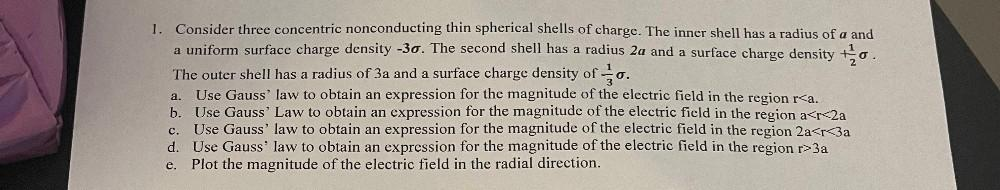Question:

# a 0. 1. Consider three concentric nonconducting thin spherical shells of charge. The inner shell has a radius of a and a uniforma 0. 1. Consider three concentric nonconducting thin spherical shells of charge. The inner shell has a radius of a and a uniform surface charge density -30. The second shell has a radius 2a and a surface charge density to The outer shell has a radius of 3a and a surface charge density of o. Use Gauss' law to obtain an expression for the magnitude of the electric field in the region r<a. b. Use Gauss' Law to obtain an expression for the magnitude of the electric field in the region a<r<2a Use Gauss' law to obtain an expression for the magnitude of the electric field in the region 2a<r<3a d. Use Gauss' law to obtain an expression for the magnitude of the electric field in the region r>3a Plot the magnitude of the electric field in the radial direction. a. C. e.